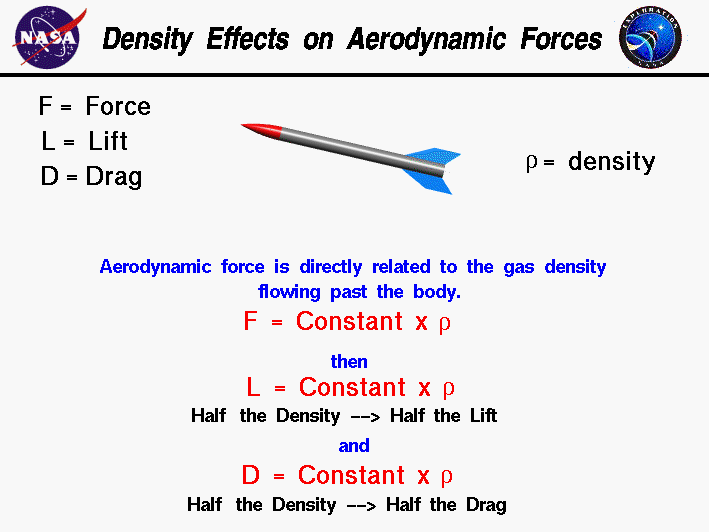+ Text Only Site
+ Non-Flash Version
+ Contact GlennAerodynamic forces are generated whenever an object moves through a liquid or gas. From Newton's second law of motion, the aerodynamic forces on the body are directly related to the change in momentum of the fluid with time. The fluid momentum is equal to the mass times the velocity of the fluid. Since the fluid is moving, defining the mass gets a little tricky. If the mass of fluid were brought to a halt, it would occupy some volume in space; and we could define its density to be the mass divided by the volume. With a little math we can show that the aerodynamic forces are directly proportional to the density of the fluid that flows by the rocket. Beginning with Newton's second law: F = d (m * V) / dt where F is the force, m is the mass, t is time, and V is the velocity. If we integrate this equation, we obtain: F = constant * V * m / t Since the fluid is moving, we must determine the mass in terms of the mass flow rate. The mass flow rate is the amount of mass passing a given point during some time interval and its units are mass/time. We can relate the mass flow rate to the density mathematically. The mass flow rate mdot is equal to the density r times the velocity times the area A through which the mass passes. mdot = m / t = r * V * A With knowledge of the mass flow rate, we can express the aerodynamic force as equal to the mass flow rate times the velocity. F = constant * V * r * V * A A quick units check: mass * length / time^2 = constant * length/time * mass/length^3 * length/time * length^2 mass * length / time^2 = mass * length/time^2 Combining the velocity dependence and absorbing the area into the constant, we find: F = constant * r * V^2 The aerodynamic force equals a constant times the density times the velocity squared. The dynamic pressure of a moving flow is equal to one half of the density times the velocity squared. Therefore, the aerodynamic force is directly proportional to the dynamic pressure qof the flow. F = constant * q where the value of this constant is different than the previous constant. During the launch of the Space Shuttle you may hear the commentator call out, "Max-Q on the vehicle." This indicates the area of maximum aerodynamic forces on the spacecraft. Lift and drag depend linearly on the density of the fluid. Halving the density halves the lift, halving the density halves the drag. The fluid density depends on the type of fluid and the depth of the fluid. In the atmosphere, air density decreases as altitude increases. The relation between altitude and density is a fairly complex exponential that has been determined by measurements in the atmosphere. A similar model has been developed for the Martian atmosphere based on satellite measurments. You can investigate the effect of density and the other factors on the flight of a rocket by using the RocketModeler III Java Applet. Guided ToursRocket Aerodynamics:Activities: Related Sites: Rocket Index Rocket Home Beginner's Guide Home+ Inspector General Hotline + Equal Employment Opportunity Data Posted Pursuant to the No Fear Act + Budgets, Strategic Plans and Accountability Reports + Freedom of Information Act + The President's Management Agenda + NASA Privacy Statement, Disclaimer, and Accessibility CertificationEditor: Tom Benson NASA Official: Tom Benson Last Updated: May 13 2021 + Contact Glenn